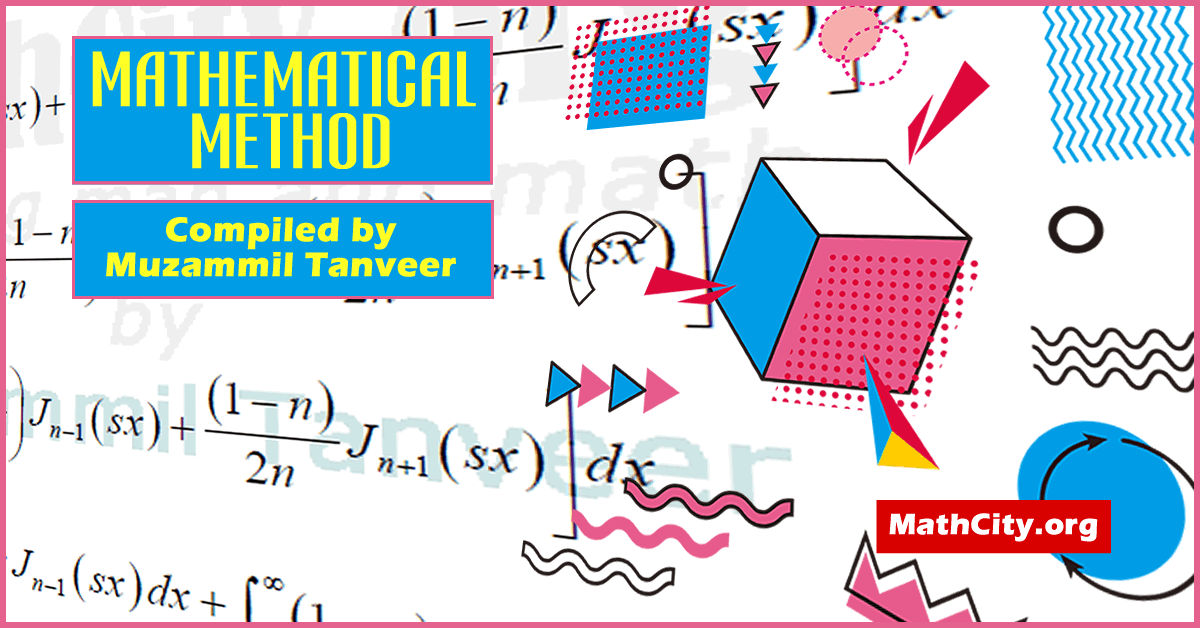# Mathematical Method by Sir Muhammad Awais Aun

These notes are provided and composed by Mr. Muzammil Tanveer. We are really very thankful to him for providing these notes and appreciates his effort to publish these notes on MathCity.orgName Mathematical Method Sir Muhammad Awais Aun Mr. Muzammil Tanveer 117 pages PDF (see Software section for PDF Reader) 1.1 MB
• Differential Equations
• Solution of a Differential Equation
• Linearly Independent Functions and the Wronskian
• Criterion for Linear Independent Functions
• Auxiliary Conditions
• Separated and Mixed Boundary Conditions
• Boundary Value Problems
• Abel’s Formula
• Eigenvalue Problems
• Self-Adjoint form of a Differential Equation
• Symmetric Operator
• Lagrange’s Identity
• Green’s Identity or Green’s formula
• Differential Equation
• Ordinary D.E (O.D.E)
• Partial D.E (P.D.E)
• Initial Condition
• Boundary Condition
• Fourier Transform
• Attenuation Property
• Even, Odd function
• Fourier Sine Transform
• Inverse Fourier
• Fourier Transformation Derivatives of a function
• Fourier Cosine Transformation
• Inverse Fourier Cosine Transformation
• Laplace Transformation
• Laplace transformation of derivative of function of f(t)
• Derivative of Laplace transformation
• Unit function
• Bessel Equation and Bessel Functions
• Basic Identities and Recurrence formula
• Parametric Bessel functions
• Transforming the independent variable for Bessel Function
• Orthogonality of Bessel functions
• Properties of Hankel transformation
• Hanker transformation of the derivation of the function f(x)
• Parseval Theorem
• Differential formula of Hankel transformation
• Green’s Identity or Green’s formula
• Green’s functions and their applications
• Green’s function for boundary value problems

Please click on View Online to see inside the PDF.

• msc/notes/mathematical-method-muzammil-tanveer